Refer to our Texas Go Math Grade 4 Answer Key Pdf to score good marks in the exams. Test yourself by practicing the problems from Texas Go Math Grade 4 Unit 2 Assessment Answer Key.

Vocabulary

• Compatible numbers
• Distributive Property
• partial product
• partial quotient
• remainder

Vocabulary

Question 1.
When a number cannot be divided evenly, the amount left over is called the _______________.
Answer:  When a number cannot be divided evenly, the amount left over is called the Remainder..

Question 2.
In the ____________ method of dividing, multiples of the divisor are subtracted from the dividend and then the quotients are added together.

In the partial quotient method of dividing, multiples of the divisor are subtracted from the dividend and then the quotients are added together.

Concepts and Skills

Estimate. Then find the sum or difference.

Question 3.
Estimate: ____________Estimate: 2.30Question 4.
Estimate: ____________Estimate : 32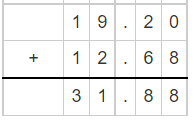Question 5.
Estimate: ____________Estimate: 4.43

Estimate. Then find the product.

Question 6.
Estimate: ____________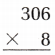Estimate: 2448Question 7.
Estimate: ____________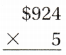Estimate: 4620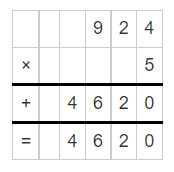Question 8.
Estimate: ____________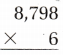Estimate:

52,788Question 9.
Estimate: ____________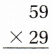Estimate: 1710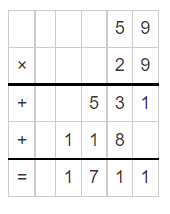Question 10.
Estimate: ____________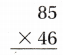Estimate:

3,910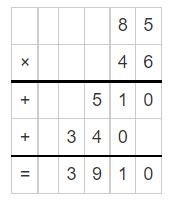Question 11.
Estimate: ____________Estimate:

2,888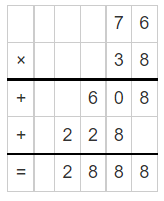Find the quotient.

Question 12.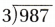Quotient: 329

Remainder: 0

Question 13.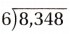Quotient: 1391

Remainder: 2

Question 14.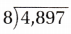Quotient = 0612

Remainder: 1

Question 15.
During September and October of 2008, the Grand Canyon National Park recorded a total of 792,426 visitors. If there were 359,396 visitors in October, how many people visited the park in September?
(A) 1,151,822
(B) 447,170
(C) 433,030
(D) 433,130

Given, The total number of people visited in September and November= 792,426

Number of people visited in October = 359,396

Now, Total – People visited in October = Number of people visited in November.

So, 792,426 – 359,396 = 433,030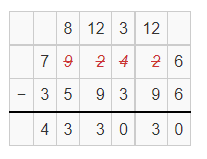Therefore, the number of visitors visited the park in September = 433,030

Question 16.
Mary has 2 bags of apples. One bag weighs 4.56 pounds and the other bag weighs 3.85 pounds. 1f she gave her sister 1.25 pounds of apples, how many pounds of apples would Mary have?
(A) 3.31 pounds
(B) 7.16 pounds
(C) 2.6 pounds
(D) 6.16 pounds

Given,

One bag weighs 4.56 pounds

Other bag weighs 3.85 pounds

Total weight of 2 bags =Also give, she gave 1.25 pounds to her sister.

So, The number of pounds of apples Mary have.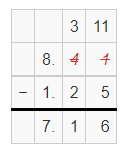Therefore, Mary have 7.16 pounds of apples.

Question 17.
At Reptile World, there are 7 times as many alligators as crocodiles. If there are 117 crocodiles in the west enclosure and 183 crocodiles in the east enclosure, how many alligators are there?
(A) 1,281
(B) 307
(C) 2,100
(D) 819

The number of crocodiles in west enclosure = 117

The number of crocodiles in east enclosure = 183

Also given, there are 7 times as many alligators as crocodiles.

Which means,

In west EnclosureIn East Enclosure,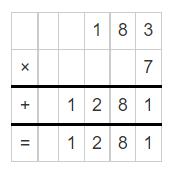Total number of alligators = 819+1281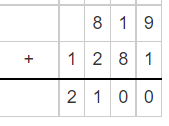Therefore, There are 2100 alligators.

Question 18.
Gardeners at The Seed Stop are planting vegetable seeds in trays. In each tray, there are 12 rows with 8 sections in each row. If they plant one seed in each section, how many plants will there be in 53 trays if all of the seeds germinate?
(A) 1,060
(B) 5,088
(C) 636
(D) 3,010

Given,

In each tray there are 12 rows and 8 sections.

Which means, 12 x 8 =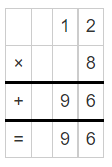Now, For 53 Trays Number of plant =

53 x 96therefore, there are 5088 plants will be there if all seeds germinate in 53 trays.

Question 19.
The correct answer to a homework problem is 2,000. Which equation could have been the homework problem?
(A) 5 × 4,000 =
(B) 50 × 400 =
(C) 50 × 40 =
(D) 50 × 4,000 =

50 × 40 =Question 20.
Jill sold 35 adult tickets for a fund raiser. An adult ticket costs $18. How much did Jill collect for the tickets? Use an area model to help you solve. (A)$615
(B) $630 (C)$740
(D) $515 Answer: The number of tickets Jill sold = 35 The cost of adult ticket =$18

Now, The amount Jill collected for the tickets =

35 x $18 = 630Therefore, Jill Collects$630 for the tickets.

Question 21.
A baby animal at the zoo eats about 30 pounds of food each day. If each baby animal eats the same amount each day, how many pounds of food do 12 baby animals eat in 5 days?
(A) 3,605 pounds
(B) 1,800 pounds
(C) 720 pounds
(D) 3,595 pounds

The amount of food a baby animal eat = 30 pounds

Total number of baby animals = 12

Now, If each baby animal eats the same amount each day,

30 x 12 =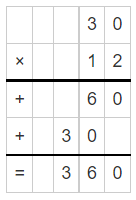Now, Number of days = 5

So, 360 x 5 =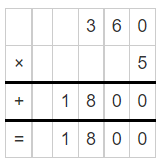Therefore, 1800 pounds of food  12 baby animals eat in 5 days.

Question 22.
A tour of the island includes 106 tourists and 8 guides. Each tour van can only hold 7 people. What is the least number of vans needed so that all of the tourists and guides go on the tour?
(A) 17
(B) 15
(C) 16
(D) 18

Number of tourists = 106

Number of guides = 8

Total number of people = 106+8 = 114

Number of people the van can hold = 7

Now, 114/7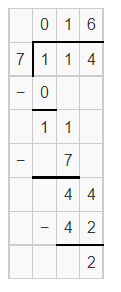Therefore, Atleast 16 vans are required so that all of the tourists and guides go on the tour.

Question 23.
Maria has 39 photos. She wants to put the same number of photos on each of 7 pages. Which of the following describes an array that would help Maria know how many photos can be on each page?
(A) an array with 5 rows of 8 squares
(B) an array with 6 rows of 7 squares
(C) an array with 4 rows of 7 squares with 11 left over squares
(D) an array with 5 rows of 7 squares with 4 leftover squares

Total number of photos = 39

Number of photos she want to put on each page = 7

Now,

39/7 =Quotient = 5

Remainder = 4

Therefore, an array with 5 rows of 7 squares with 4 leftover squares

Question 24.
Mr. Bigham has 8 months to save $456 to buy a bike. He wants to save an equal amount each month. How much must he save each month to have enough to buy the bike? Use an area model to help you solve. (A)$57
(B) $47 (C)$3,648
(D) $67 Answer: The cost of the bike =$456

Number of months = 8Therefore, he have to save $57 to buy the bike. Question 25. There are 156 math students in the 4th grade. There are 6 math classes of equal size. How many math students are in each class? (A) 200 (B) 45 (C) 26 (D) 52 Answer: Total number of students = 156 Number of classes = 6 Now, 156/6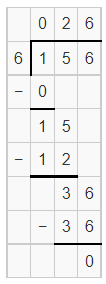Therefore, there are 26 students in each class. Question 26. Allison’s Bakery will donate$1,200 worth of baked goods to a school event. The school wants to order 12 loaves of holiday bread, 18 dozen biscuits, 12 dozen bagels, and 14 dozen multigrain rolls. Is the cost of the baked goods under the $1,200 donation limit? Explain how you found your answer. If yes, what could the school add to the order? If no, what could the school remove from the order?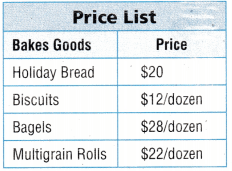Answer: The total amount of the money =$1200

The cost of Holiday bread = $20 Number of holiday bread = 12 Now,The cost of Biscuits =$12

Number of biscuits = 18

Now,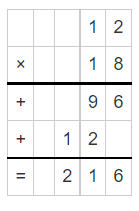The cost of Bagles = $28 Number of bagles = 12 Now,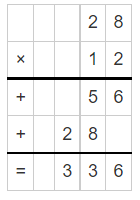The cost of multigrain rolls=$22

Number of multigrain rolls = 14Now, The total of items =

240 + 216 + 336+308 = 1108

Which means, the school did not exceed the limit.

So, 1200 – 1108 = 92

92 can be divided as  4 holiday bread and 1 biscuit/dozen

Therefore, they can buy 4 holiday bread and 1 biscuit/dozen to reach the limit.

Scroll to Top